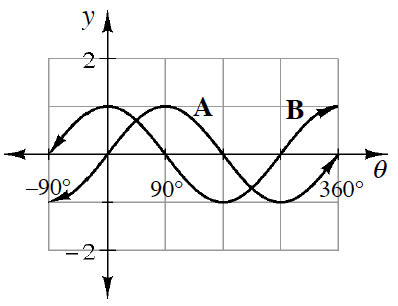### Home > INT3 > Chapter 9 > Lesson 9.1.4 > Problem9-55

9-55.

Daniel sketches the graphs at right for $y = \text{sin}\left(θ\right)$ and $y = \text{cos}\left(θ\right)$.

Unfortunately, he forgets to label the graphs, and now he cannot remember which graph goes with which equation. Explain to Daniel how he can tell (and remember!) which graph is $y = \text{sin}\left(θ\right)$ and which is $y = \text{cos}\left(θ\right)$.

Look at the $y$-intercepts. What is $\text{cos}\left(0^\circ\right)$? $\text{sin}\left(0^\circ\right)$?